Next: 9 Restrictions on Changes Up: 8.3 Binary Table Extension Previous: 8.3.3 Data Sequence

## 8.3.4 Data Display

Character data are encoded under format code Aw. If the character datum has length less than or equal to w, it is represented on output right-justified in a string of w characters. If the character datum has length greater than w, the first w characters of the datum are represented on output in a string of w characters. Character data are not surrounded by single or double quotation marks unless those marks are themselves part of the data value.

Logical data are encoded under format code Lw. Logical data are represented on output with the character T for true or F for false right justified in a blank-filled string of w characters. A null value may be represented by a completely blank string of w characters.

Integer data (including bit X and byte B type fields) are encoded under format codes Iw.m, Bw.m, Ow.m, and Zw.m. The default value of m is one and the .m'' is optional. The first letter of the code specifies the number base for the encoding with I for decimal (10), B for binary (2), O for octal (8), and Z for hexadecimal (16). Hexadecimal format uses the upper-case letters A through F to represent decimal values 10 through 15. The output field consists of w characters containing zero or more leading blanks followed by a minus if the internal datum is negative followed by the magnitude of the internal datum in the form of an unsigned integer constant in the specified number base with only as many leading zeros as are needed to have at least m numeric digits. Note that m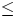w is allowed if all values are positive, but m < w is required if any values are negative. If the number of digits required to represent the integer datum exceeds w, then the output field consists of a string of w asterisk (*) characters.

Real data are encoded under format codes Fw.d, Ew.dEe, Dw.dEe, ENw.d, and ESw.d. In all cases, the output is a string of w characters including the decimal point, any sign characters, and any exponent including the exponent's indicators, signs, and values. If the number of digits required to represent the real datum exceeds w, then the output field consists of a string of w asterisk (*) characters. In all cases, d specifies the number of digits to appear to the right of the decimal point. The F format code output field consists of w-d-1 characters containing zero or more leading blanks followed by a minus if the internal datum is negative followed by the absolute magnitude of the internal datum in the form of an unsigned integer constant. These characters are followed by a decimal point (.'') and d characters giving the fractional part of the internal datum, rounded by the normal rules of arithmetic to d fractional digits. For the E and D format codes, an exponent is taken such that the fraction 0.1|datum|/10exponent<1.0. The fraction (with appropriate sign) is output with an F format of width w-e-2 characters with d characters after the decimal followed by an E or D followed by the exponent as a signed e+1 character integer with leading zeros as needed. The default value of e is 2 when the Ee portion of the format code is omitted. If the exponent value will not fit in e+1 characters but will fit in e+2 then the E (or D) is omitted and the wider field used. If the exponent value will not fit (with a sign character) in e+2characters, then the entire w-character output field is filled with asterisks (*). The ES format code is processed in the same manner as the E format code except that the exponent is taken so that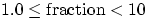. The EN format code is processed in the same manner as the E format code except that the exponent is taken to be an integer multiple of 3 and so that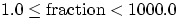. All real format codes have number base 10. There is no difference between E and D format codes on input other than an implication with the latter of greater precision in the internal datum.

The Gw.dEe format code may be used with data of any type. For data of type integer, logical, or character, it is equivalent to Iw, Lw, or Aw, respectively. For data of type real, it is equivalent to an F format (with different numbers of characters after the decimal) when that format will accurately represent the value and is equivalent to an E format when the number (in absolute value) is either very small or very large. Specifically, for real values outside the range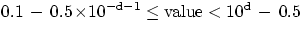, it is equivalent to Ew.dEe. For real values within the above range, it is equivalent to F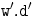followed by 2+e blanks, where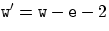and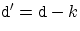for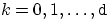if the real datum value lies in the range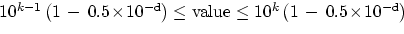.

Complex data are encoded with any of the real data formats as described above. The same format is used for the real and imaginary parts. It is recommended that the 2 values be separated by a comma and enclosed in parentheses with a total field width of 2w+3.Next: 9 Restrictions on Changes Up: 8.3 Binary Table Extension Previous: 8.3.3 Data Sequence

5/13/1999